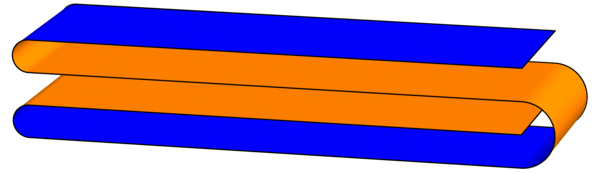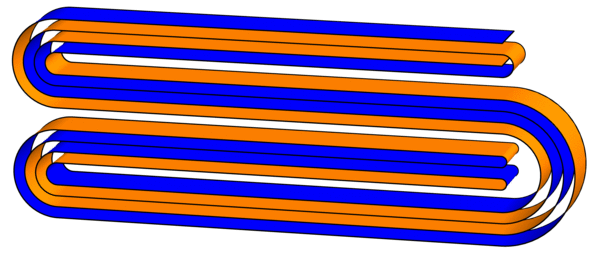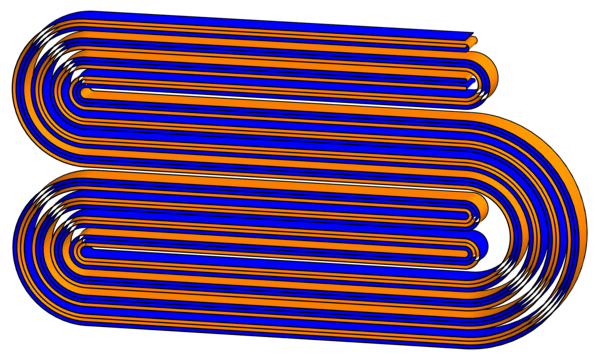# Thue-Morse-igami

Here’s a simple origami experiment to try. Start with a long strip of paper with different colors on the two sides—e.g., Orange (O) on one side, Indigo (I) on the other. Now fold the strip in quarters to form a flat “S-shape” as illustrated here (creases are drawn slightly rounded for ease of viewing):As you look through the four layers from top to bottom, write down the colors you encounter, in order: the first layer shows Indigo (I), the second layer has Orange (O), and so on. The full order is IOOI, which looks suspiciously like the start of the Thue-Morse sequence. (This sequence was introduced last week.)

To continue the experiment, treat this figure as a single strip and fold the same flat S-shape again. There will be 16 layers down the middle:Sure enough, the order of colors encountered is IOOI OIIO OIIO IOOI, which is the first 16 digits of the Thue-Morse sequence. This pattern will continue forever (or at least until the paper gets too thick to fold): every time you fold another S-shape, you quadruple the number of digits of the Thue-Morse sequence (can you see why?). Try to fold a third iteration! It looks like this (click for larger image):But wait, there’s more! Now that we have these creased strips, what happens when we unfold them? Specifically, follow the existing creases and lay out the strips so that each crease makes a 90-degree angle. What shapes do we get? Surprisingly, they exactly fill triangular grids of squares:Can you figure out why this pattern continues?

If we consider digits instead of letters or words, then numbers such as 676, 100020001, and 12345678987654321 are palindromes. These are in fact quite special palindromes, as they are all squares of integers: $$26^2 = 676$$, $$111111111^2 = 12345678987654321$$, and $$10001^2 = 100020001$$. Are there infinitely many palindromic squares? Yes: the last example above hints that $$10\ldots01^2 = 10\ldots020\ldots01$$ for however many 0s you put in the middle. By contrast, if we ask for $$n^5$$ to be palindromic (or any higher power), then no solutions are currently known!
You might ask for other types of palindromic numbers, such as palindromic primes. A few examples include 7 (this is a palindrome!), 101, 19391, and 1000000008000000001. Are there infinitely many? We don’t know. But the largest palindromic prime currently known is $$10^{200000} + 47960506974 \cdot 10^{99995} + 1$$, according to Wikipedia.